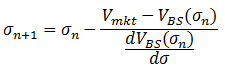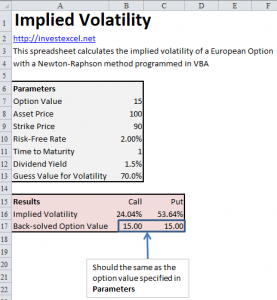# Calculate Implied Volatility with VBA

This article offers VBA code and an Excel spreadsheet to calculate the implied volatility of an option. This parameter is often compared to the historical volatility of the underlying asset to determine if the price of an option represents good value.

Implied volatility is the volatility estimated from the option price, asset price, strike price risk-free-rate, time to maturity and dividend yield. Calculating implied volatility needs iterative solution methods. Several technique are commonly used; one method uses Excel’s Goal Seek functionality, while other approaches use bisection or Newton-Raphson iteration.

The theory behind the Newton-Raphson method for finding the root of an equation is well documented. The approach gives the following equation to calculate the implied volatility of an option.• Vmkt is the market price of the option
• VBS is the option price given by the Black-Scholes equation
• σ is the volatility

## Implied Volatility with Newton-Raphson Iteration

This VBA function calculates the price of a European option with the Black-Scholes equation.

```Function EuropeanOption(CallOrPut, S, K, v, r, T, q)
Dim d1 As Double, d2 As Double, nd1 As Double, nd2 As Double
Dim nnd1 As Double, nnd2 As Double```
```d1 = (Log(S / K) + (r - q + 0.5 * v ^ 2) * T) / (v * Sqr(T))
d2 = (Log(S / K) + (r - q - 0.5 * v ^ 2) * T) / (v * Sqr(T))
nd1 = Application.NormSDist(d1)
nd2 = Application.NormSDist(d2)
nnd1 = Application.NormSDist(-d1)
nnd2 = Application.NormSDist(-d2)```
```If CallOrPut = "Call" Then
EuropeanOption = S * Exp(-q * T) * nd1 - K * Exp(-r * T) * nd2
Else
EuropeanOption = -S * Exp(-q * T) * nnd1 + K * Exp(-r * T) * nnd2
End If
End Function```

This VBA function calculates the implied volatility of a European option with Newton-Raphson iteration.

```Function ImpliedVolatility(CallOrPut, S, K, r, T, q, OptionValue, guess)
Dim epsilon As Double, dVol As Double, vol_1 As Double
Dim i As Integer, maxIter As Integer, Value_1 As Double, vol_2 As Double
Dim Value_2 As Double, dx As Double

dVol = 0.00001
epsilon = 0.00001
maxIter = 100
vol_1 = guess
i = 1
Do
Value_1 = EuropeanOption(CallOrPut, S, K, vol_1, r, T, q)
vol_2 = vol_1 - dVol
Value_2 = EuropeanOption(CallOrPut, S, K, vol_2, r, T, q)
dx = (Value_2 - Value_1) / dVol
If Abs(dx) < epsilon Or i = maxIter Then Exit Do
vol_1 = vol_1 - (OptionValue - Value_1) / dx
i = i + 1
Loop
ImpliedVolatility = vol_1
End Function```
• S is the asset price
• CallOrPut is either “Call” or “Put”
• K is the strike price
• r is the risk-free rate
• q is the dividend yield
• v is the volatility
• epsilon is a calculation tolerance
• maxIter is the maximum allowable number of iterations

This Excel spreadsheet implements the two VBA functions given above for a sample option.As a check, the implied volatility is used to calculate the option price using the Black-Scholes equation. This should be equal to the option value specified in the Parameters section.

## 5 thoughts on “Calculate Implied Volatility with VBA”

1. Mike says:

in your formula, the denominator is supposed to be – Vega
and not + vega as it is the derivative of the function to minimize wrt sigma
?

2. JimC says:

Hi. In your variable definitions, ‘S’ is the asset price, not the strike price. Thanks for publishing this!

3. Vi says:

Hi, thank you so much for your post, I really appreciate it. However I have some problem when apply this into Garman Kohlhagen model which is basically Black-Scholes for foreign exchange option. The implied volatility, some are negative (I do it on multiple cells) and they all are far from the result I got when using Goal Seek. The Black Scholes option value thus is not the same as the market’s option price. Please help !!!